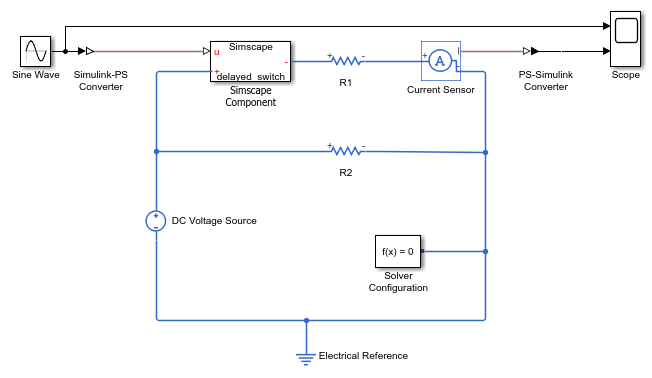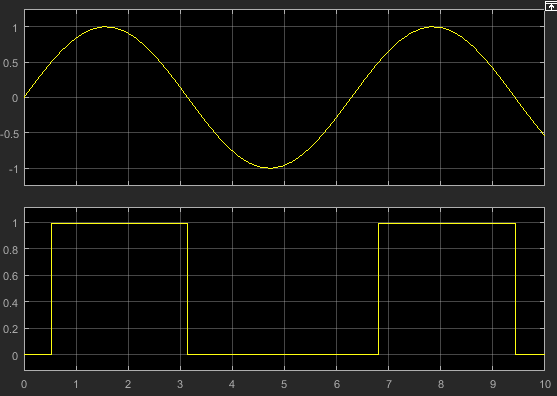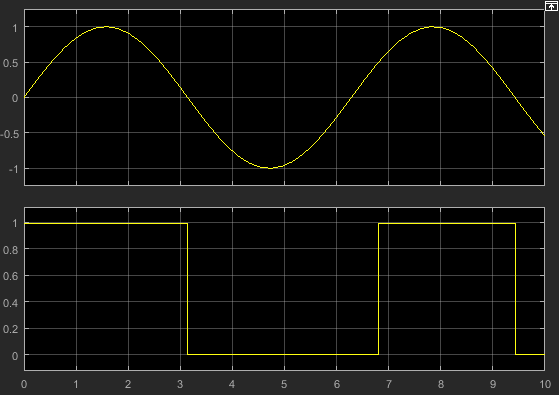## Switch with Hysteresis

The Switch block in the Simscape™ Foundation library implements a switch controlled by an external physical signal. The block uses an `if-else` statement. If the external physical signal at the control port is greater than the threshold, then the switch is closed, otherwise the switch is open.

This example implements a switch with hysteresis applied to the switching threshold level. The hysteresis acts to prevent rapid spurious switching when the control signal is noisy.

The switch has two distinct operating modes, shown in the diagram. If the external physical signal at the control port is greater than the upper threshold, then the switch is closed. If the signal is lower than the lower threshold, the switch is open.The following component implements the logic in the diagram by using a mode chart.

```component delayed_switch % Switch with Hysteresis inputs u = { 0.0, '1' }; end nodes p = foundation.electrical.electrical; % + n = foundation.electrical.electrical; % -:right end parameters R_closed = { 0.01, 'Ohm' }; % Closed resistance R_closed G_open = { 1e-8, '1/Ohm' }; % Open conductance G_open T_closed = { 0.5, '1' }; % Upper threshold T_open = { 0, '1' }; % Lower threshold InitMode = switching.open; % Initial Mode end variables i = { 0, 'A' }; % Current v = { 0, 'V' }; % Voltage end branches i : p.i -> n.i; end % Validate parameter values equations assert( T_closed >= T_open, 'Upper threshold must be higher than Lower threshold' ); end modecharts(ExternalAccess = observe) m1 = modechart modes mode CLOSED equations v == p.v - n.v; v == i*R_closed; end end mode OPEN equations v == p.v - n.v; v == i/G_open; end end end transitions CLOSED -> OPEN : u < T_open; OPEN -> CLOSED : u > T_closed; end initial OPEN : InitMode <= 0; end end end end ```

The mode chart `m1` defines two modes, `CLOSED` and `OPEN`. Each mode has an `equations` section that lists all the applicable equations. The `transitions` section defines the transitions between the operating modes, based on predicate conditions:

• The switch transitions from `CLOSED` to `OPEN` when the control signal falls below the lower threshold, `T_open`.

• The switch transitions from `OPEN` to `CLOSED` when the control signal rises above the upper threshold, `T_closed`.

The `initial` section specifies the initial operating mode, based on a predicate condition:

• If the predicate is true (that is, the Initial Mode parameter value is less than or equal to 0), then the `OPEN` mode is active at the start of simulation.

• If the predicate is not true, then the `CLOSED` mode (the first mode listed in the `modes` section) is active at the start of simulation.

Note

The Initial Mode parameter uses an enumeration:

```classdef switching < int32 enumeration open (0) closed (1) end methods(Static) function map = displayText() map = containers.Map; map('open') = 'Switch is open'; map('closed') = 'Switch is closed'; end end end ```

For the component to work as described, this enumeration needs to be in a separate `switching.m` file. The file can be located either on the MATLAB® path or in a package imported into the component. In general, enumerations are very useful in mode charts, because they let you specify a discrete set of acceptable parameter values. For more information, see Enumerations.

To verify the correct component behavior, deploy it in a Simscape Component block. Create a simple test model, as shown, with all the blocks using the default parameter values.Simulate the model with the default values.The Initial Mode parameter value is ```Switch is open```. This enumerated value evaluates to 0, which makes the predicate in the `initial` section true. Therefore, at the start of simulation the switch is open and no current flows through the resistor R1. When the control signal value reaches 0.5 (the Upper threshold parameter value), the switch closes and the current through the branch, based on the other parameter values, is 1A. When the control signal falls below 0 (the Lower threshold parameter value), the switch opens.

Now change the Initial Mode parameter value to ```Switch is closed``` and simulate the model. The enumerated value evaluates to 1, the predicate condition in the `initial` section is no longer true, and therefore the first mode listed in the `modes` section is active. At the start of simulation, the switch is closed, and it stays closed until the control signal falls below 0.